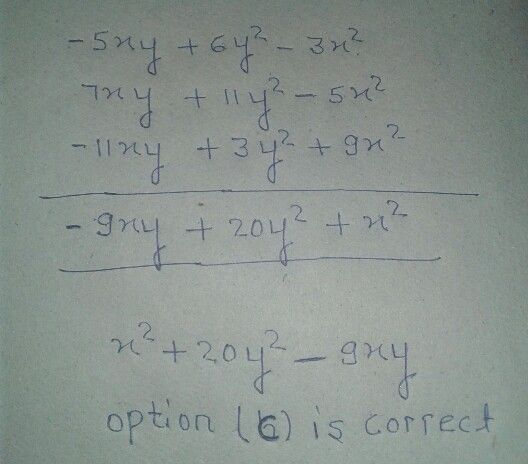Symbol
ProblemThe sum of $-5xy+6y^{2}-3x^{2}$ $11y^{2}+7xy-5x^{2}$ and $9x^{2}-11xy+3y^{2}is$ a) $\right)$ $x^{2}-9xy-20y^{2}$ b) $\right)-x^{2}+9xy+20y^{2}$ c) $\right)$ $x^{2}-9xy+20y^{2}$ d) $1\right)$ $x^{2}+9xy-20y^{2}$